• 详细来讲讲梯度下降算法原理，感受数学和程序的魅力吧！！


博文目录
1. 概述2. 梯度下降算法2.1 场景假设2.2 梯度下降2.2.1 微分2.2.2 梯度
2.3 数学解释2.3.1 α2.3.2 梯度要乘以一个负号

3. 实例3.1 单变量函数的梯度下降3.2 多变量函数的梯度下降
4. 代码实现4. 1 场景分析4. 2 代码
5. 小结

1. 概述
2. 梯度下降算法
2.1 场景假设
梯度下降法的基本思想可以类比为一个下山的过程。 假设这样一个场景：一个人被困在山上，需要从山上下来(找到山的最低点，也就是山谷)。但此时山上的浓雾很大，导致可视度很低；因此，下山的路径就无法确定，必须利用自己周围的信息一步一步地找到下山的路。这个时候，便可利用梯度下降算法来帮助自己下山。怎么做呢，首先以他当前的所处的位置为基准，寻找这个位置最陡峭的地方，然后朝着下降方向走一步，然后又继续以当前位置为基准，再找最陡峭的地方，再走直到最后到达最低处；同理上山也是如此，只是这时候就变成梯度上升算法了2.2 梯度下降
梯度下降的基本过程就和下山的场景很类似。
首先，我们有一个可微分的函数。这个函数就代表着一座山。我们的目标就是找到这个函数的最小值，也就是山底。根据之前的场景假设，最快的下山的方式就是找到当前位置最陡峭的方向，然后沿着此方向向下走，对应到函数中，就是找到给定点的梯度 ，然后朝着梯度相反的方向，就能让函数值下降的最快！因为梯度的方向就是函数之变化最快的方向(在后面会详细解释) 所以，我们重复利用这个方法，反复求取梯度，最后就能到达局部的最小值，这就类似于我们下山的过程。而求取梯度就确定了最陡峭的方向，也就是场景中测量方向的手段。那么为什么梯度的方向就是最陡峭的方向呢？接下来，我们从微分开始讲起：
2.2.1 微分
看待微分的意义，可以有不同的角度，最常用的两种是：
函数图像中，某点的切线的斜率函数的变化率 几个微分的例子：
1.单变量的微分，函数只有一个变量时

d

(

x

2

)

d

x

=

2

x

\frac{d(x^2)}{dx}=2x

d

(

−

2

y

5

)

d

y

=

−

10

y

4

\frac{d(-2y^5)}{dy}=-10y^4

d

(

5

−

θ

)

2

d

θ

=

−

2

(

5

−

θ

)

\frac{d(5-\theta )^2}{d\theta}=-2(5-\theta)

2.多变量的微分，当函数有多个变量的时候，即分别对每个变量进行求微分

∂

∂

x

(

x

2

y

2

)

=

2

x

y

2

\frac{\partial}{\partial x}(x^2y^2) = 2xy^2

∂

∂

y

(

−

2

y

5

+

z

2

)

=

−

10

y

4

\frac{\partial}{\partial y}(-2y^5+z^2) = -10y^4

∂

∂

θ

2

(

5

θ

1

+

2

θ

2

−

12

θ

3

)

=

2

\frac{\partial}{\partial \theta_{2}}(5\theta_{1} + 2\theta_{2} - 12\theta_{3}) = 2

∂

∂

θ

2

(

0.55

−

(

5

θ

1

+

2

θ

2

−

12

θ

3

)

)

=

−

2

\frac{\partial}{\partial \theta_{2}}(0.55 - (5\theta_{1} + 2\theta_{2} - 12\theta_{3})) = -2

2.2.2 梯度
梯度实际上就是多变量微分的一般化。 下面这个例子：

J

(

Θ

)

=

0.55

−

(

5

θ

1

+

2

θ

2

−

12

θ

3

)

J(\Theta ) = 0.55 - (5\theta_{1} + 2\theta_{2} - 12\theta_{3})

▽

J

(

Θ

)

=

<

∂

J

∂

θ

1

,

∂

J

∂

θ

2

,

∂

J

∂

θ

3

>

=

(

−

5

,

−

2

,

12

)

\triangledown J(\Theta ) = \left < \frac{\partial J}{\partial \theta_{1}}, \frac{\partial J}{\partial \theta_{2}},\frac{\partial J}{\partial \theta_{3}} \right > =(-5,-2,12)

我们可以看到，梯度就是分别对每个变量进行微分，然后用逗号分割开，梯度是用<>包括起来，说明梯度其实一个向量。
梯度是微积分中一个很重要的概念，之前提到过梯度的意义
在单变量的函数中，梯度其实就是函数的微分，代表着函数在某个给定点的切线的斜率在多变量函数中，梯度是一个向量，向量有方向，梯度的方向就指出了函数在给定点的上升最快的方向
这也就说明了为什么我们需要千方百计的求取梯度！我们需要到达山底，就需要在每一步观测到此时最陡峭的地方，梯度就恰巧告诉了我们这个方向。梯度的方向是函数在给定点上升最快的方向，那么梯度的反方向就是函数在给定点下降最快的方向，这正是我们所需要的。所以我们只要沿着梯度的方向一直走，就能走到局部的最低点！
2.3 数学解释
首先给出数学公式：

Θ

1

=

Θ

0

+

α

▽

J

(

Θ

)

→

e

v

a

l

u

a

t

e

d

a

t

Θ

0

{\color{Red} \Theta^1} = {\color{Blue} \Theta^0} + {\color{Green} \alpha} {\color{Purple} \triangledown J(\Theta)}\rightarrow evaluated at \Theta^0

此公式的意义是：J是关于Θ的一个函数，我们当前所处的位置为Θ0点，要从这个点走到J的最小值点，也就是山底。首先我们先确定前进的方向，也就是梯度的反向，然后走一段距离的步长，也就是α，走完这个段步长，就到达了Θ1这个点！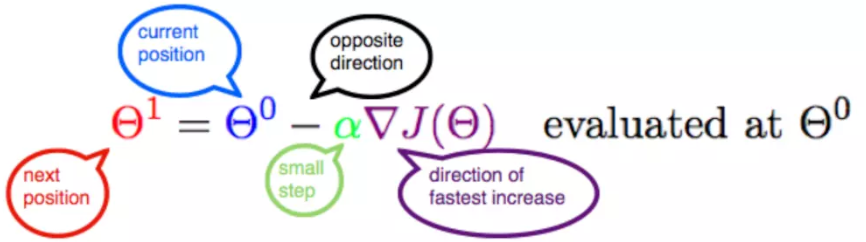2.3.1 α
α在梯度下降算法中被称作为学习率或者步长，意味着我们可以通过α来控制每一步走的距离，以保证不要步子跨的太大扯着蛋，哈哈，其实就是不要走太快，错过了最低点。同时也要保证不要走的太慢，导致太阳下山了，还没有走到山下。所以α的选择在梯度下降法中往往是很重要的！α不能太大也不能太小，太小的话，可能导致迟迟走不到最低点，太大的话，会导致错过最低点！
2.3.2 梯度要乘以一个负号
梯度前加一个负号，就意味着朝着梯度相反的方向前进！我们在前文提到，梯度的方向实际就是函数在此点上升最快的方向！而我们需要朝着下降最快的方向走，自然就是负的梯度的方向，所以此处需要加上负号；那么如果时上坡，也就是梯度上升算法，当然就不需要添加负号了。
3. 实例
我们已经基本了解了梯度下降算法的计算过程，那么我们就来看几个梯度下降算法的小实例，首先从单变量的函数开始，然后介绍多变量的函数。
3.1 单变量函数的梯度下降
我们假设有一个单变量的函数

J

(

θ

)

=

θ

2

J(\theta) = \theta^2

函数的微分，直接求导就可以得到

J

′

(

θ

)

=

2

θ

J'(\theta) = 2\theta

初始化，也就是起点，起点可以随意的设置，这里设置为1

θ

0

=

1

\theta^0 = 1

学习率也可以随意的设置，这里设置为0.4

α

=

0.4

\alpha = 0.4

根据梯度下降的计算公式

Θ

1

=

Θ

0

+

α

▽

J

(

Θ

)

→

e

v

a

l

u

a

t

e

d

a

t

Θ

0

{\color{Red} \Theta^1} = {\color{Blue} \Theta^0} + {\color{Green} \alpha} {\color{Purple} \triangledown J(\Theta)}\rightarrow evaluated at \Theta^0

我们开始进行梯度下降的迭代计算过程：

θ

0

=

1

\theta^0 = 1

θ

1

=

θ

0

−

α

∗

J

′

(

θ

0

)

=

1

−

0.4

∗

2

=

0.2

\theta^1 = \theta^0 - \alpha*J'(\theta^0)=1 - 0.4*2 = 0.2

θ

2

=

θ

1

−

α

∗

J

′

(

θ

1

)

=

0.2

−

0.4

∗

0.4

=

0.04

\theta^2 = \theta^1 - \alpha*J'(\theta^1)= 0.2 - 0.4*0.4=0.04

θ

3

=

0.008

\theta^3 = 0.008

θ

4

=

0.0016

\theta^4 = 0.0016

如图，经过四次的运算，也就是走了四步，基本就抵达了函数的最低点，也就是山底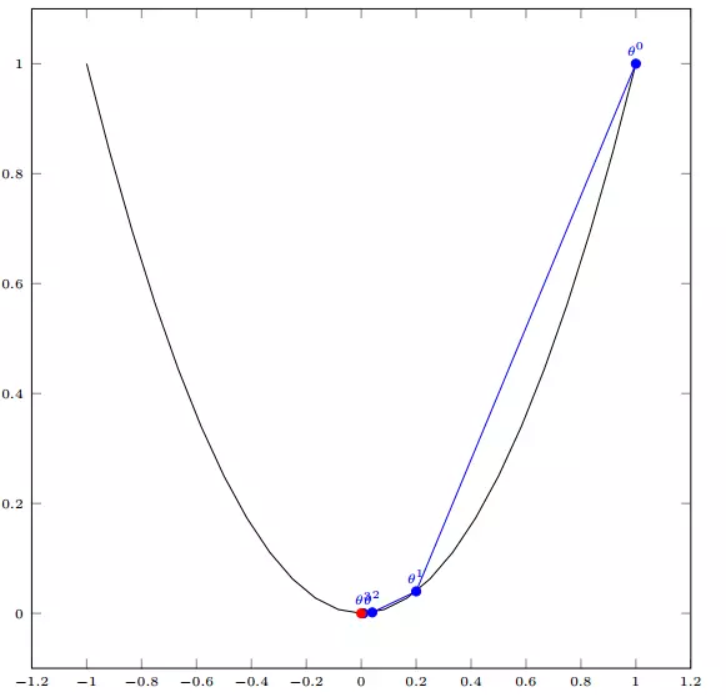3.2 多变量函数的梯度下降
我们假设有一个目标函数

J

(

Θ

)

=

θ

1

2

+

θ

2

2

J(\Theta) = \theta_{1}^2 + \theta_{2}^2

现在要通过梯度下降法计算这个函数的最小值。我们通过观察就能发现最小值其实就是 (0，0)点。但是接下来，我们会从梯度下降算法开始一步步计算到这个最小值！ 我们假设初始的起点为：

Θ

0

=

(

1

,

3

)

\Theta^0 = (1, 3)

初始的学习率为：

α

=

0.1

\alpha = 0.1

函数的梯度为：

▽

J

(

Θ

)

=

<

2

θ

1

,

2

θ

2

>

\triangledown J(\Theta ) = \left < 2\theta_{1},2\theta_{2} \right >

进行多次迭代：

Θ

0

=

(

1

,

3

)

\Theta^0 = (1, 3)

Θ

1

=

Θ

0

−

α

▽

J

(

Θ

)

=

(

1

,

3

)

−

0.1

∗

(

2

,

6

)

=

(

0.8

,

2.4

)

\Theta^1 = \Theta^0 - \alpha\triangledown J(\Theta ) = (1,3) - 0.1*(2, 6)=(0.8, 2.4)

Θ

2

=

(

0.8

,

2.4

)

−

0.1

∗

(

1.6

,

4.8

)

=

(

0.64

,

1.92

)

\Theta^2 = (0.8, 2.4) - 0.1*(1.6, 4.8)=(0.64, 1.92)

Θ

3

=

(

0.5124

,

1.536

)

\Theta^3 =(0.5124, 1.536)

Θ

4

=

(

0.4096

,

1.228800000000001

)

\Theta^4 =(0.4096, 1.228800000000001)

⋮

\vdots

Θ

10

=

(

0.1073741824000003

,

0.32212254720000005

)

\Theta^{10} =(0.1073741824000003, 0.32212254720000005)

⋮

\vdots

Θ

50

=

(

1.141798154164342

e

−

05

,

3.42539442494306

e

−

05

)

\Theta^{50} =(1.141798154164342e^{-05}, 3.42539442494306e^{-05})

⋮

\vdots

Θ

100

=

(

1.6296287810675902

e

−

10

,

4.8888886343202771

e

−

10

)

\Theta^{100} =(1.6296287810675902e^{-10}, 4.8888886343202771e^{-10})

我们发现，已经基本靠近函数的最小值点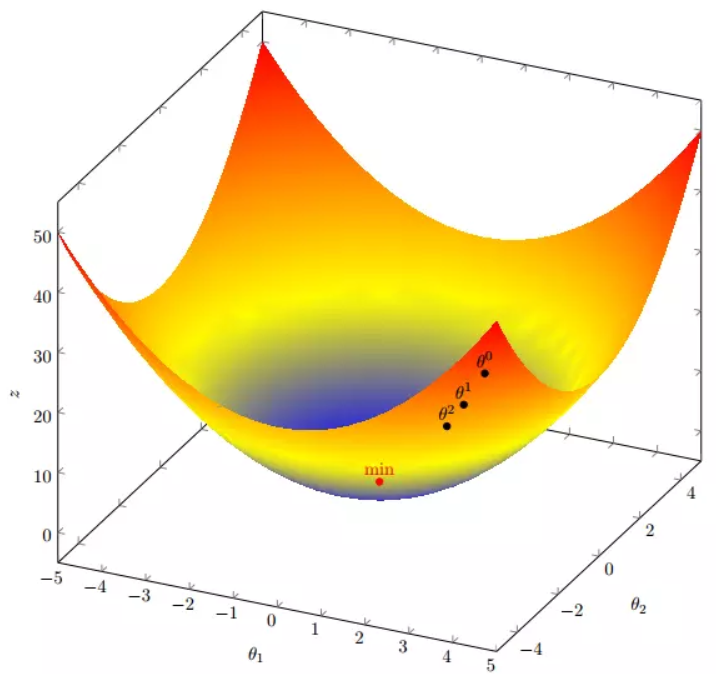4. 代码实现
4. 1 场景分析
下面我们将用python实现一个简单的梯度下降算法。场景是一个简单的线性回归的例子：假设现在我们有一系列的点，如下图所示：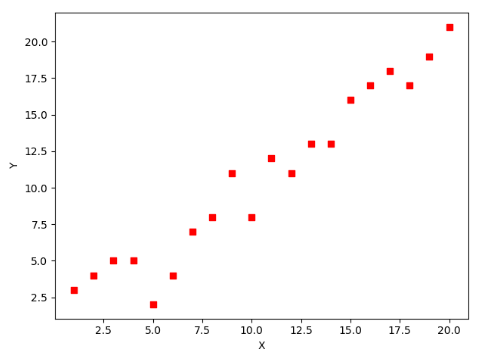我们将用梯度下降法来拟合出这条直线！
首先，我们需要定义一个代价函数，在此我们选用均方误差代价函数（也称平方误差代价函数）

J

(

Θ

)

=

1

2

m

∑

i

=

1

m

(

h

θ

(

x

(

i

)

)

−

y

(

i

)

)

2

J(\Theta) = \frac{1}{2m}\sum_{i=1}^{m}(h_{\theta}(x^{(i)})-y^{(i)})^2

此公式中
m是数据集中数据点的个数，也就是样本数½是一个常量，这样是为了在求梯度的时候，二次方乘下来的2就和这里的½抵消了，自然就没有多余的常数系数，方便后续的计算，同时对结果不会有影响y 是数据集中每个点的真实y坐标的值，也就是类标签h 是我们的预测函数（假设函数），根据每一个输入x，根据Θ 计算得到预测的y值，即

h

Θ

(

x

(

i

)

)

=

Θ

0

+

Θ

1

x

1

(

i

)

h_{\Theta}(x^{(i)}) = \Theta_{0} + \Theta_{1}x_{1}^{(i)}

我们可以根据代价函数看到，代价函数中的变量有两个，所以是一个多变量的梯度下降问题，求解出代价函数的梯度，也就是分别对两个变量进行微分

▽

J

(

Θ

)

=

<

δ

J

δ

Θ

0

,

δ

J

δ

Θ

1

>

\triangledown J(\Theta ) = \left < \frac{\delta J}{\delta \Theta_{0}}, \frac{\delta J}{\delta \Theta_{1}} \right >

δ

J

δ

Θ

0

=

1

m

∑

i

=

1

m

(

h

Θ

(

x

(

i

)

)

−

y

(

i

)

)

\frac{\delta J}{\delta \Theta_{0}} = \frac{1}{m}\sum_{i=1}^{m}(h_{\Theta}(x^{(i)})-y^{(i)})

δ

J

δ

Θ

1

=

1

m

∑

i

=

1

m

(

h

Θ

(

x

(

i

)

)

−

y

(

i

)

)

x

1

(

i

)

\frac{\delta J}{\delta \Theta_{1}} = \frac{1}{m}\sum_{i=1}^{m}(h_{\Theta}(x^{(i)})-y^{(i)})x_{1}^{(i)}

明确了代价函数和梯度，以及预测的函数形式。我们就可以开始编写代码了。但在这之前，需要说明一点，就是为了方便代码的编写，我们会将所有的公式都转换为矩阵的形式，python中计算矩阵是非常方便的，同时代码也会变得非常的简洁。 为了转换为矩阵的计算，我们观察到预测函数的形式

h

Θ

(

x

(

i

)

)

=

Θ

0

+

Θ

1

x

(

i

)

h_{\Theta}(x^{(i)}) = \Theta_{0} + \Theta_{1}x^{(i)}

我们有两个变量，为了对这个公式进行矩阵化，我们可以给每一个点x增加一维，这一维的值固定为1，这一维将会乘到Θ0上。这样就方便我们统一矩阵化的计算

(

x

1

(

i

)

,

y

(

i

)

)

→

(

x

0

(

i

)

,

x

1

(

i

)

,

y

(

i

)

)

w

i

t

h

x

0

(

i

)

=

1

∀

i

(x_{1}^{(i)},y^{(i)})\rightarrow (x_{0}^{(i)},x_{1}^{(i)},y^{(i)}) with x_{0}^{(i)} = 1 \forall _{i}

然后我们将代价函数和梯度转化为矩阵向量相乘的形式

J

(

Θ

)

=

1

2

m

(

X

Θ

−

y

⃗

)

T

(

X

Θ

−

y

⃗

)

J(\Theta) = \frac{1}{2m}(X\Theta - \vec{y})^{T}(X\Theta - \vec{y})

▽

J

(

Θ

)

=

1

m

X

T

(

X

Θ

−

y

⃗

)

)

\triangledown J(\Theta) = \frac{1}{m}X^{T}(X\Theta - \vec{y}))

4. 2 代码
首先，我们需要定义数据集和学习率
#!/usr/bin/env python3
# -*- coding: utf-8 -*-
# @Time    : 2019/1/21 21:06
# @Author  : Arrow and Bullet
# @Software: PyCharm
# @Blog    ：https://blog.csdn.net/qq_41800366

from numpy import *

# 数据集大小 即20个数据点
m = 20
# x的坐标以及对应的矩阵
X0 = ones((m, 1))  # 生成一个m行1列的向量，也就是x0，全是1
X1 = arange(1, m+1).reshape(m, 1)  # 生成一个m行1列的向量，也就是x1，从1到m
X = hstack((X0, X1))  # 按照列堆叠形成数组，其实就是样本数据
# 对应的y坐标
y = np.array([
3, 4, 5, 5, 2, 4, 7, 8, 11, 8, 12,
11, 13, 13, 16, 17, 18, 17, 19, 21
]).reshape(m, 1)
# 学习率
alpha = 0.01

接下来我们以矩阵向量的形式定义代价函数和代价函数的梯度
# 定义代价函数
def cost_function(theta, X, Y):
diff = dot(X, theta) - Y  # dot() 数组需要像矩阵那样相乘，就需要用到dot()
return (1/(2*m)) * dot(diff.transpose(), diff)

# 定义代价函数对应的梯度函数
diff = dot(X, theta) - Y
return (1/m) * dot(X.transpose(), diff)

最后就是算法的核心部分，梯度下降迭代计算
# 梯度下降迭代
theta = array([1, 1]).reshape(2, 1)
theta = theta - alpha * gradient
return theta

print('optimal:', optimal)
print('cost function:', cost_function(optimal, X, Y))

当梯度小于1e-5时，说明已经进入了比较平滑的状态，类似于山谷的状态，这时候再继续迭代效果也不大了，所以这个时候可以退出循环！ 运行代码，计算得到的结果如下：
print('optimal:', optimal)  # 结果 [[0.51583286][0.96992163]]
print('cost function:', cost_function(optimal, X, Y))  # 1.014962406233101

通过matplotlib画出图像，
# 根据数据画出对应的图像
def plot(X, Y, theta):
import matplotlib.pyplot as plt
ax = plt.subplot(111)  # 这是我改的
ax.scatter(X, Y, s=30, c="red", marker="s")
plt.xlabel("X")
plt.ylabel("Y")
x = arange(0, 21, 0.2)  # x的范围
y = theta + theta*x
ax.plot(x, y)
plt.show()

plot(X1, Y, optimal)

所拟合出的直线如下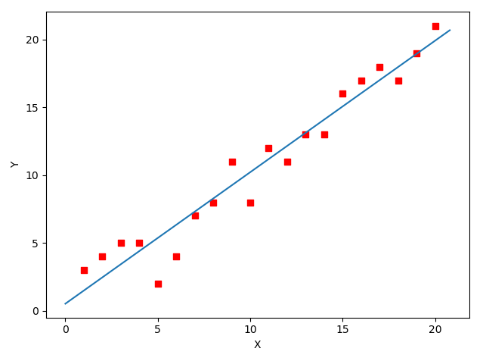全部代码如下，大家有兴趣的可以复制下来跑一下看一下结果：
#!/usr/bin/env python3
# -*- coding: utf-8 -*-
# @Time    : 2019/1/21 21:06
# @Author  : Arrow and Bullet
# @Software: PyCharm
# @Blog    ：https://blog.csdn.net/qq_41800366

from numpy import *

# 数据集大小 即20个数据点
m = 20
# x的坐标以及对应的矩阵
X0 = ones((m, 1))  # 生成一个m行1列的向量，也就是x0，全是1
X1 = arange(1, m+1).reshape(m, 1)  # 生成一个m行1列的向量，也就是x1，从1到m
X = hstack((X0, X1))  # 按照列堆叠形成数组，其实就是样本数据
# 对应的y坐标
Y = array([
3, 4, 5, 5, 2, 4, 7, 8, 11, 8, 12,
11, 13, 13, 16, 17, 18, 17, 19, 21
]).reshape(m, 1)
# 学习率
alpha = 0.01

# 定义代价函数
def cost_function(theta, X, Y):
diff = dot(X, theta) - Y  # dot() 数组需要像矩阵那样相乘，就需要用到dot()
return (1/(2*m)) * dot(diff.transpose(), diff)

# 定义代价函数对应的梯度函数
diff = dot(X, theta) - Y
return (1/m) * dot(X.transpose(), diff)

# 梯度下降迭代
theta = array([1, 1]).reshape(2, 1)
theta = theta - alpha * gradient
return theta

print('optimal:', optimal)
print('cost function:', cost_function(optimal, X, Y))

# 根据数据画出对应的图像
def plot(X, Y, theta):
import matplotlib.pyplot as plt
ax = plt.subplot(111)  # 这是我改的
ax.scatter(X, Y, s=30, c="red", marker="s")
plt.xlabel("X")
plt.ylabel("Y")
x = arange(0, 21, 0.2)  # x的范围
y = theta + theta*x
ax.plot(x, y)
plt.show()

plot(X1, Y, optimal)

5. 小结
至此，就基本介绍完了梯度下降法的基本思想和算法流程，并且用python实现了一个简单的梯度下降算法拟合直线的案例！ 最后，我们回到文章开头所提出的场景假设: 这个下山的人实际上就代表了反向传播算法，下山的路径其实就代表着算法中一直在寻找的参数Θ，山上当前点的最陡峭的方向实际上就是代价函数在这一点的梯度方向，场景中观测最陡峭方向所用的工具就是微分 。在下一次观测之前的时间就是有我们算法中的学习率α所定义的。 可以看到场景假设和梯度下降算法很好的完成了对应！
本文部分内容来自一位前辈，非常感谢分享！谢谢！
展开全文• 文章目录2.5 梯度下降法介绍学习目标1 全梯度下降算法（FG）2 随机梯度下降算法（SG）3 小批量梯度下降算法（mini-batch）4 随机平均梯度下降算法（SAG）5 小结 2.5 梯度下降法介绍 学习目标 知道全梯度下降算法的...


文章目录
2.5 梯度下降法介绍学习目标1 全梯度下降算法（FG）2 随机梯度下降算法（SG）3 小批量梯度下降算法（mini-batch）4 随机平均梯度下降算法（SAG）5 小结

2.5 梯度下降法介绍
学习目标
知道全梯度下降算法的原理知道随机梯度下降算法的原理知道随机平均梯度下降算法的原理知道小批量梯度下降算法的原理
常见的梯度下降算法有：
它们都是为了正确地调节权重向量，通过为每个权重计算一个梯度，从而更新权值，使目标函数尽可能最小化。其差别在于样本的使用方式不同。
1 全梯度下降算法（FG）
计算训练集所有样本误差，对其求和再取平均值作为目标函数。
权重向量沿其梯度相反的方向移动，从而使当前目标函数减少得最多。
因为在执行每次更新时，我们需要在整个数据集上计算所有的梯度，所以批梯度下降法的速度会很慢，同时，批梯度下降法无法处理超出内存容量限制的数据集。
批梯度下降法同样也不能在线更新模型，即在运行的过程中，不能增加新的样本。
其是在整个训练数据集上计算损失函数关于参数θ的梯度：2 随机梯度下降算法（SG）
由于FG每迭代更新一次权重都需要计算所有样本误差，而实际问题中经常有上亿的训练样本，故效率偏低，且容易陷入局部最优解，因此提出了随机梯度下降算法。
其每轮计算的目标函数不再是全体样本误差，而仅是单个样本误差，即每次只代入计算一个样本目标函数的梯度来更新权重，再取下一个样本重复此过程，直到损失函数值停止下降或损失函数值小于某个可以容忍的阈值。
此过程简单，高效，通常可以较好地避免更新迭代收敛到局部最优解。其迭代形式为其中，x(i)表示一条训练样本的特征值，y(i)表示一条训练样本的标签值

但是由于，SG每次只使用一个样本迭代，若遇上噪声则容易陷入局部最优解。
3 小批量梯度下降算法（mini-batch）
小批量梯度下降算法是FG和SG的折中方案,在一定程度上兼顾了以上两种方法的优点。
每次从训练样本集上随机抽取一个小样本集，在抽出来的小样本集上采用FG迭代更新权重。
被抽出的小样本集所含样本点的个数称为batch_size，通常设置为2的幂次方，更有利于GPU加速处理。
特别的，若batch_size=1，则变成了SG；若batch_size=n，则变成了FG.其迭代形式为4 随机平均梯度下降算法（SAG）
在SG方法中，虽然避开了运算成本大的问题，但对于大数据训练而言，SG效果常不尽如人意，因为每一轮梯度更新都完全与上一轮的数据和梯度无关。
随机平均梯度算法克服了这个问题，在内存中为每一个样本都维护一个旧的梯度，随机选择第i个样本来更新此样本的梯度，其他样本的梯度保持不变，然后求得所有梯度的平均值，进而更新了参数。
如此，每一轮更新仅需计算一个样本的梯度，计算成本等同于SG，但收敛速度快得多。
拓展阅读： 梯度下降法算法比较和进一步优化
5 小结
全梯度下降算法（FG）【知道】
在进行计算的时候,计算所有样本的误差平均值,作为我的目标函数 随机梯度下降算法（SG）【知道】
每次只选择一个样本进行考核 小批量梯度下降算法（mini-batch）【知道】
选择一部分样本进行考核 随机平均梯度下降算法（SAG）【知道】
会给每个样本都维持一个平均值,后期计算的时候,参考这个平均值 
展开全文机器学习
• 梯度下降，依照所给数据，判断函数，随机给一个初值w，之后通过不断更改，一步步接近原函数的方法。更改的过程也就是根据梯度不断修改w的过程。 以简单的一元函数为例 原始数据为 x_data = [1.0,2.0,3.0] y_data...
梯度下降算法
梯度下降，依照所给数据，判断函数，随机给一个初值w，之后通过不断更改，一步步接近原函数的方法。更改的过程也就是根据梯度不断修改w的过程。
以简单的一元函数为例
原始数据为
x_data = [1.0,2.0,3.0]
y_data = [2.0,4.0,6.0]
因此我们设置函数为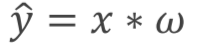对于该函数，我们的w是未知的，因此如何根据xy的数据，获取到正确的w值就是梯度下降的目标。
首先我们要先给定一个随机w值，这个值可以是任何数，我们的算法就会根据我们所计算的cost函数，判断偏离正确数据有多大，之后根据梯度，对w进行更新，直到cost为0，我们也就获取到正确的w值。
cost函数，也就是根据自己的模拟量，算出的结果与原函数所给数据的差值的平方
cost函数的表示为（所求的是N个数据的平均cost）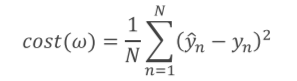cost函数对w的求导为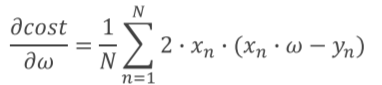每次更改的过程就是不断更新w，（a也就是每次w改变的步长，用a乘以w的偏导）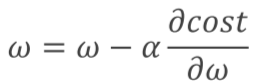最终当cost为0时，就基本可以保证函数模拟的是正确的。
梯度下降的python实现
x_data = [1.0,2.0,3.0]
y_data = [2.0,4.0,6.0]
w = 1.0

def forward(x):
return x*w
def cost(xs,ys):
cost = 0
for x,y in zip(xs,ys):
y_pred = forward(x)
cost+=(y_pred - y) **2
return cost / len(xs)
'''求平均的cost大小'''

for x,y in zip(xs,ys):
'''求平均的梯度大小'''

print("Predict (before training)",4,forward(4))
for epoch in range(100):
cost_val = cost(x_data,y_data)
print('EPOCH:',epoch,'w=',w,'loss=',cost_val)
print("Predict(after training)",4,forward(4))
'''对下一个数据进行预测的结果'''
由于梯度算法，是对所有的差值求平均，因此，很有可能困在局部最优解之中。举个例子，一个人在下山的过程中，不断找周围的最低点，有的人可以直接下山，但是有的人在半山腰山遇到一个水池，对这个水池来说，四周都比它高，因此，就会被困在这个水池中，没法下山。因此解决办法就是随机梯度下降。
随机梯度下降
采用随机梯度下降，相较于求平均的cost，采用随机的loss函数，也就是每次只取一个值，还是上个例子，当这个人困在水池中是，突然随机出现一个点，告诉你你的周围还有更低点，你就可以走出水池，然后重新走向下山的道路
求w的导数函数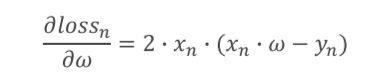loss函数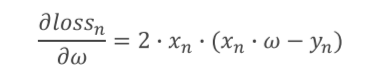对于x，y参数，不像梯度下降的cost函数要遍历x，y的原数据，而只是使用当前的数据x,y即可
随机梯度下降的python实现
x_data = [1.0,2.0,3.0]
y_data = [2.0,4.0,6.0]
w = 1.0

def forward(x):
return x*w

def loss(x,y):
y_pred = forward(x)
return(y_pred-y)**2

return 2*x*y*(x*w-y)

print('Predict(before training)',4,forward(4))
for epoch in range(100):
for x,y in zip(x_data,y_data):
'''相比于梯度下降需要一次对所有数据求取平均值，随机梯度下降需要进行两次循环,
在第二次循环中，对于每个数据都要单独求取一个梯度'''
'''分别对三个数据求取梯度'''
l = loss(x,y)
print("progress:",epoch,"w=",w,"loss",l)
print("Prediect (after training)",4,forward(4))

展开全文python
• 文章目录线性回归学习目标2.5 梯度下降法介绍1 全梯度下降算法（FG）2 随机梯度下降算法（SG）3 小批量梯度下降算法（mini-bantch）4 随机平均梯度下降算法（SAG）5 算法比较6 梯度下降优化算法(拓展) 学习目标 ...
线性回归

文章目录
线性回归学习目标
2.5 梯度下降法介绍1 全梯度下降算法（FG）2 随机梯度下降算法（SG）3 小批量梯度下降算法（mini-bantch）4 随机平均梯度下降算法（SAG）5 算法比较6 梯度下降优化算法(拓展)

学习目标
掌握线性回归的实现过程应用LinearRegression或SGDRegressor实现回归预测知道回归算法的评估标准及其公式知道过拟合与欠拟合的原因以及解决方法知道岭回归的原理及与线性回归的不同之处应用Ridge实现回归预测应用joblib实现模型的保存与加载
2.5 梯度下降法介绍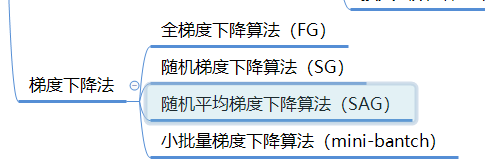上一节中给大家介绍了最基本的梯度下降法实现流程，常见的梯度下降算法有：
它们都是为了正确地调节权重向量，通过为每个权重计算一个梯度，从而更新权值，使目标函数尽可能最小化。其差别在于样本的使用方式不同。
1 全梯度下降算法（FG）
计算训练集所有样本误差，对其求和再取平均值作为目标函数。
权重向量沿其梯度相反的方向移动，从而使当前目标函数减少得最多。
因为在执行每次更新时，我们需要在整个数据集上计算所有的梯度，所以批梯度下降法的速度会很慢，同时，批梯度下降法无法处理超出内存容量限制的数据集。
批梯度下降法同样也不能在线更新模型，即在运行的过程中，不能增加新的样本。
其是在整个训练数据集上计算损失函数关于参数θ的梯度：2 随机梯度下降算法（SG）
由于FG每迭代更新一次权重都需要计算所有样本误差，而实际问题中经常有上亿的训练样本，故效率偏低，且容易陷入局部最优解，因此提出了随机梯度下降算法。
其每轮计算的目标函数不再是全体样本误差，而仅是单个样本误差，即每次只代入计算一个样本目标函数的梯度来更新权重，再取下一个样本重复此过程，直到损失函数值停止下降或损失函数值小于某个可以容忍的阈值。
此过程简单，高效，通常可以较好地避免更新迭代收敛到局部最优解。其迭代形式为每次只使用一个样本迭代，若遇上噪声则容易陷入局部最优解。

其中，x(i)表示一条训练样本的特征值，y(i)表示一条训练样本的标签值

但是由于，SG每次只使用一个样本迭代，若遇上噪声则容易陷入局部最优解。
3 小批量梯度下降算法（mini-bantch）
小批量梯度下降算法是FG和SG的折中方案,在一定程度上兼顾了以上两种方法的优点。
每次从训练样本集上随机抽取一个小样本集，在抽出来的小样本集上采用FG迭代更新权重。
被抽出的小样本集所含样本点的个数称为batch_size，通常设置为2的幂次方，更有利于GPU加速处理。
特别的，若batch_size=1，则变成了SG；若batch_size=n，则变成了FG.其迭代形式为4 随机平均梯度下降算法（SAG）
在SG方法中，虽然避开了运算成本大的问题，但对于大数据训练而言，SG效果常不尽如人意，因为每一轮梯度更新都完全与上一轮的数据和梯度无关。
随机平均梯度算法克服了这个问题，在内存中为每一个样本都维护一个旧的梯度，随机选择第i个样本来更新此样本的梯度，其他样本的梯度保持不变，然后求得所有梯度的平均值，进而更新了参数。
如此，每一轮更新仅需计算一个样本的梯度，计算成本等同于SG，但收敛速度快得多。
5 算法比较
以下6幅图反映了模型优化过程中四种梯度算法的性能差异。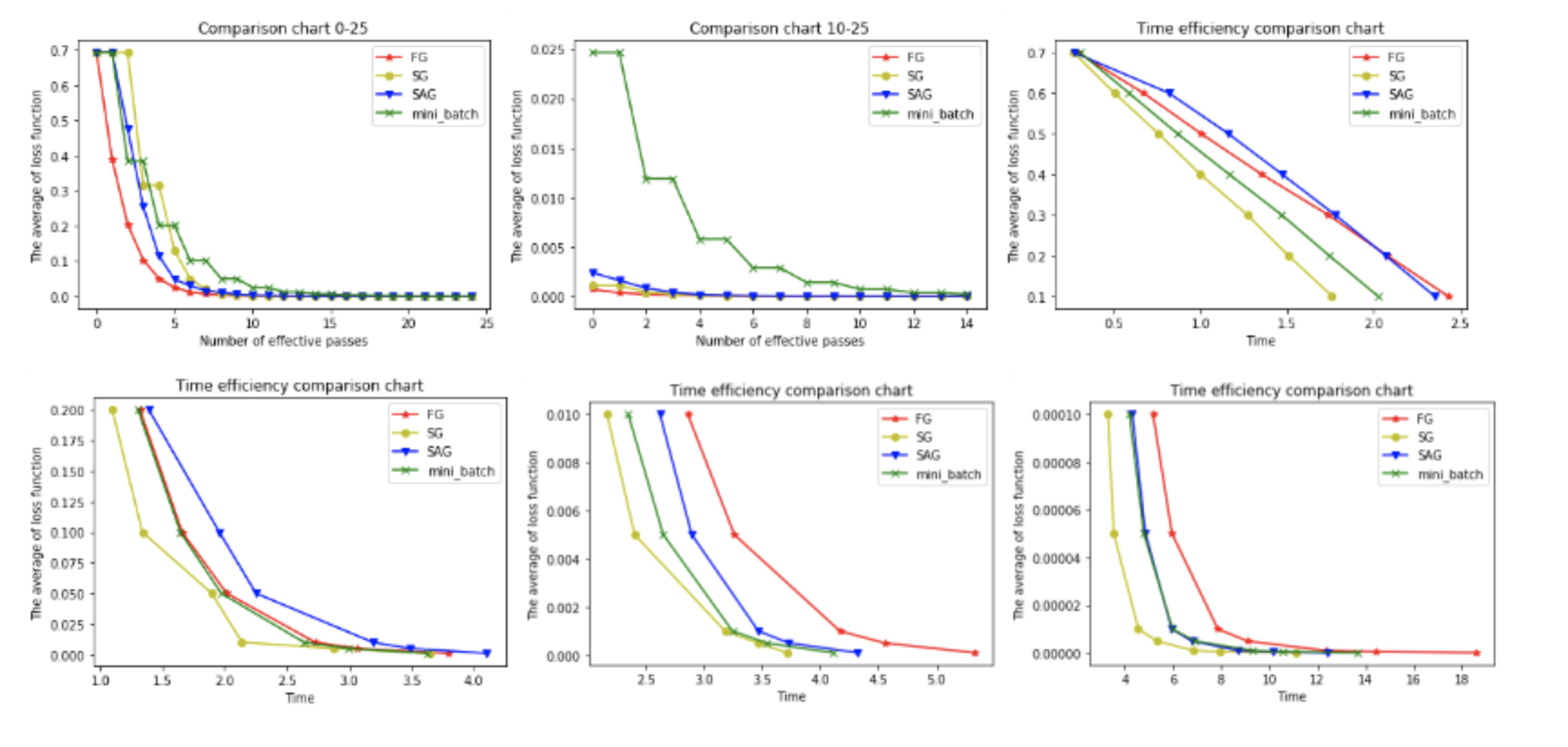在图1和图2中，横坐标代表有效迭代次数，纵坐标代表平均损失函数值。图1反映了前25次有效迭代过程中平均损失函数值的变化情况，为了便于观察，图2放大了第10次到25次的迭代情况。
从图1中可以看到，四种梯度算法下，平均损失函数值随迭代次数的增加而减少。FG的迭代效率始终领先，能在较少的迭代次数下取得较低的平均损失函数值。FG与SAG的图像较平滑，这是因为这两种算法在进行梯度更新时都结合了之前的梯度；SG与mini-batch的图像曲折明显，这是因为这两种算法在每轮更新梯度时都随机抽取一个或若干样本进行计算，并没有考虑到之前的梯度。
从图2中可以看到**虽然四条折现的纵坐标虽然都趋近于0，但SG和FG较早，mini-batch最晚。**这说明如果想使用mini-batch获得最优参数，必须对其进行较其他三种梯度算法更多频次的迭代。
在图3，4，5，6中，横坐标表示时间，纵坐标表示平均损失函数值。
从图3中可以看出使用四种算法将平均损失函数值从0.7降到0.1最多只需要2.5s，由于本文程序在初始化梯度时将梯度设为了零，故前期的优化效果格外明显。其中SG在前期的表现最好，仅1.75s便将损失函值降到了0.1，虽然SG无法像FG那样达到线性收敛，但在处理大规模机器学习问题时，为了节约时间成本和存储成本，可在训练的一开始先使用SG，后期考虑到收敛性和精度可改用其他算法。
从图4，5，6可以看出，随着平均损失函数值的不断减小，SG的性能逐渐反超FG，FG的优化效率最慢，即达到相同平均损失函数值时FG所需要的时间最久。
综合分析六幅图我们得出以下结论：
（1**）FG方法由于它每轮更新都要使用全体数据集，故花费的时间成本最多，内存存储最大。**
（2）SAG在训练初期表现不佳，优化速度较慢。这是因为我们常将初始梯度设为0，而SAG每轮梯度更新都结合了上一轮梯度值。
（3）综合考虑迭代次数和运行时间，SG表现性能都很好，能在训练初期快速摆脱初始梯度值，快速将平均损失函数降到很低。但要注意，在使用SG方法时要慎重选择步长，否则容易错过最优解。
（4）mini-batch结合了SG的“胆大”和FG的“心细”，从6幅图像来看，它的表现也正好居于SG和FG二者之间。在目前的机器学习领域，mini-batch是使用最多的梯度下降算法，正是因为它避开了FG运算效率低成本大和SG收敛效果不稳定的缺点。
6 梯度下降优化算法(拓展)
以下这些算法主要用于深度学习优化
动量法
其实动量法(SGD with monentum)就是SAG的姐妹版SAG是对过去K次的梯度求平均值SGD with monentum 是对过去所有的梯度求加权平均 Nesterov加速梯度下降法
参考链接：https://blog.csdn.net/google19890102/article/details/69942970
展开全文python 神经网络 机器学习 人工智能
• 随机梯度下降法 数学原理Minimising cost function is the holy grail of machine learning. Gradient descent is our master key to all cost minimisation problems. But gradient descent is slow. It uses ...深度学习 机器学习 python 神经网络 人工智能
• 梯度下降法是一种机器学习中常用的优化算法，用来找到一个函数(f)的参数(系数)的值，使成本函数(cost)最小。当参数不能解析计算时(如使用线性代数)，并且必须通过优化算法搜索时，它是最佳选择。批梯度下降法batch...
• I . 梯度下降 Gradient Descent 简介 ( 梯度下降过程 | 梯度下降方向 ) II . 梯度下降 示例说明 ( 单个参数 ) III . 梯度下降 示例说明 ( 多个参数 ) IV ....V ....VI ....VII . 随机梯度下降法 VIII . 小批量梯度下降法数据挖掘 梯度下降 损失函数
• ## 随机梯度下降算法

千次阅读 2019-03-08 14:57:52
主要内容：提供不同算法原理以及效果直观展示，并希望读者能够在实际问题中更合理的选用梯度下降类算法。 目录： 1.简介梯度下降法 2.随机梯度下降 3.随机梯度下降的问题与...4.随机梯度下降的优化算法（主要内容）
• 日萌社 人工智能AI：Keras PyTorch MXNet ...梯度下降法的基本思想可以类比为一个下山的过程。 假设这样一个场景： 一个人被困在山上，需要从山上下来(i.e. 找到山的最低点，也就是山谷)。但此时山上的浓雾很大...人工智能python
• 二、随机梯度下降法（Stochastic Gradient Descent，SGD） 和批量梯度下降法原理类似，区别在于求梯度时没有用所有的N个样本的数据，而是仅仅选取一个样本来求梯度 优点：速度快 缺点：准确度低 三、小批量梯度下降...梯度下降 批量梯度下降 小批量梯度下降
• backpropagation backpropagation解决的核心问题损失函数c与w,b求偏导，(c为cost(w,b)) 整体来说，分两步 1.z=w*a’+b 2.a=sigmoid(z) 其中，a’表示上一层的输出值，a表示当前该层的输出值 1，输入x，正向的更新一...
• 梯度下降法随机梯度下降算法、批量梯度下降 梯度下降：梯度下降就是我上面的推导，要留意，在梯度下降中，对于θ的更新，所有的样本都有贡献，也就是参与调整θ 其计算得到的是一个标准梯度。因而理论上来说一次...机器学习
• 无论是机器学习（Machine Learning）...下面我们逐个介绍梯度下降法（GD）、随机梯度下降法（SGD）和随机平均梯度下降法（SAGD）。先来看梯度下降法的基本思想。 基本原理 如果抛开具体场景，从数学抽象角度来看...梯度下降法 随机平均梯度下降法
• 针对现有分布式计算环境下随机梯度下降算法存在效率性与私密性矛盾的问题，提出一种 MapReduce框架下满足差分隐私的随机梯度下降算法。该算法基于MapReduce框架，将数据随机分配到各个Map节点并启动Map分任务独立...机器学习 MapReduce 差分隐私保护 拉普拉斯机制
• 梯度下降和随机梯度下降是机器学习中最常用的算法之一。关于其具体的原理这里不多做介绍，网络上可以很方便的找到。例如可以参考博客：http://blog.csdn.net/woxincd/article/details/7040944 scala代码实现如下：...机器学习 大数据分析 scala 数据挖掘
• 批量梯度下降、随机梯度下降、小批量梯度下降的python实现 之前写线性回归那一块内容的时候，发过手写二元线性回归梯度下降的博，由于基于方程的写法过于复杂，于是有了这一篇基于矩阵的梯度下降实现~批量梯度下降 小批量梯度下降 python
• 阅读目录批量梯度下降法BGD随机梯度下降法SGD小批量梯度下降法MBGD总结 批量梯度下降法BGD 随机梯度下降法SGD 小批量梯度下降法MBGD 总结 在应用机器学习算法时，我们常采用梯度下降法来对才用的算法进行训练。梯度...
• 本文以二维线性拟合为例，介绍批量梯度下降法、随机梯度下降法、小批量梯度下降法三种方法，求解拟合的线性模型参数。 需要拟合的数据集是 $(X_1, y_1), (X_2, y_2)..., (X_n, y_n)$，其中$X^i=(x_1^i, x_2^i)$，...
• 在机器学习领域，体梯度下降算法分为三种 - 批量梯度下降算法（BGD，...- 随机梯度下降算法（SGD，Stochastic gradient descent algorithm） - 小批量梯度下降算法（MBGD，Mini-batch gradient descent algorithm）机器学习
• 批量梯度下降法就是在计算代价函数的时候会用到所有的样本数目，比如当权值都为1的时候会得到一个假设函数好h(x),然后运用所有...随机梯度下降法，是在计算代价函数的时候只运用一个样本，进行更新权值。计算量小 ...深度学习 神经网络 机器学习
• ## 随机梯度下降原理

千次阅读 2017-08-06 19:07:09
梯度下降（GD）是最小化风险函数、损失函数的一种常用方法，随机梯度下降和批量梯度下降是两种迭代求解思路，下面从公式和实现的角度对两者进行分析，如有哪个方面写的不对，希望网友纠正。 下面的h(x)是要拟合...
• https://www.jianshu.com/p/a20e11416a25 看的三种算法的完整版
• 梯度下降算法作为一种最优化的算法，简单来说就是沿着梯度下降的方向寻求一个函数的最小值。有关梯度下降原理，推荐...本文主要把批量梯度下降随机梯度下降算法在线性回归中的应用用代码的形式展示出来，供初学...最优化算法 机器学习
• 梯度下降法原理可以参考：斯坦福机器学习第一讲。 我实验所用的数据是100个二维点。 如果梯度下降算法不能正常运行，考虑使用更小的步长(也就是学习率)，这里需要注意两点： 1）对于足够小的, 能保证在每一步都......